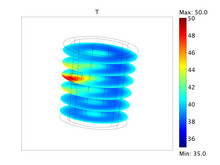# Articles techniques et présentations

#### Optimal PDE Control Using COMSOL Multiphysics

I. Neitzel, U. Prüfert, and T. Slawig

Technische Universität Berlin / SPP 1253
Technische Universität Berlin / Matheon
Excellence Cluster The Future Ocean, Algorithmic Optimal Control - Oceanic CO2-Uptake/SPP 1253
Published in 2008

An optimal control problem (OCP) is studied to a PDE of elliptic type as well as state constraints. The resulting optimality system contains two PDEs, one algebraic equation and the so called complementary slackness conditions, i.e. dual products between function spaces. At this point, different regularization techniques come into use. In this paper we introduce a Barrier method as one possible way to regularize state constraints, which leads to an easily implementable path-following algorithm. To illustrate this method, we solve first a constructed problem with a known solution. Here, we can verify the rate of convergence of the path-following method. Second, a simplified hyperthermia problem in 3D is solved by using COMSOL Multiphysics.